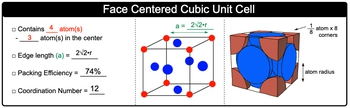Start typing, then use the up and down arrows to select an option from the list.## General Chemistry

Learn the toughest concepts covered in Chemistry with step-by-step video tutorials and practice problems by world-class tutors

13. Liquids, Solids & Intermolecular Forces

# Face Centered Cubic Unit Cell

Face Centered Cubic Unit Cell contains 4 atoms in total.

Face Centered Cubic Unit Cell
1
concept

## Face Centered Cubic Unit Cell Concept 11m
Play a video:
the face centered cubic unit cell is composed of a cube with an atom in each corner and three atoms on the faces. Now, if we took all of this into consideration, that means that a face centered cubic unit cell has a total of four atoms per one unit cell. And again three of them are in the center. If we take a look here at the image, remember each one of these red spheres is shared by neighboring unit cells. Each one represents 1/8 of an atom. And since there are eight corners, 18 times eight means that this unit cell, one Atom of it comes from the corners. And then here we have these six spheres in the center. This unit sell shares half of each of those spheres. Alright, so we'd say here because of that, that gives us another three atoms and that's how we come up with our total of four total atoms for our face centered cubic unit self. Now the edge length because there's a small little gap here. Our edge link will not be just simply to our becomes now to times square to two times are. And remember as our complexity increases both our packing efficiency as well as our coordination number will increase here are packing efficiency becomes 74% And our coordination number now is 12. Now, these are the important ideas and key values associated with any type of face centered cubic unit cells. So keep them in mind anytime we're dealing with this particular type of unit cells2
example

## Face Centered Cubic Unit Cell Example 151s
Play a video:
silver dumps a face centered cubic unit cell structure illustrate how the number of atoms per unit cell for that silver atoms are obtained. Alright so remember we have eight corners to our cube, So each one is 1/8 of an atom and we have eight corners involved. So 18 top times eight equals one adam. Also remember that on each face we have half of a sphere and there are six faces faces to acute. That's time six. So that's gonna give us three atoms when we add them together. That explains why a face centered cubic unit cell is four atoms per one unit itself. So this is our atoms to unit cell ratio. It's a 4-1 ratio.
3
Problem

Aluminum has a face-centered cubic unit structure and a density of 2.716 g/cm3. Calculate the edge length of the unit cell.

4
Problem

An element crystallizes in a face-centered cubic lattice and has a density of 1.45 g/cm3. The edge of its unit cell is 4.52×10–8 cm. How many atoms are in each unit cell?

5
Problem

An element crystallizes in a face-centered cubic lattice and has a density of 1.45 g/cm3. The edge of its unit cell is 4.52×10-8 cm. Calculate the atomic mass for the element.

6
Problem

Zinc selenide, ZnSe, crystallizes in a face-centered cubic unit cell has a density of 5.42 g/cm3. What is the volume of a unit cell?